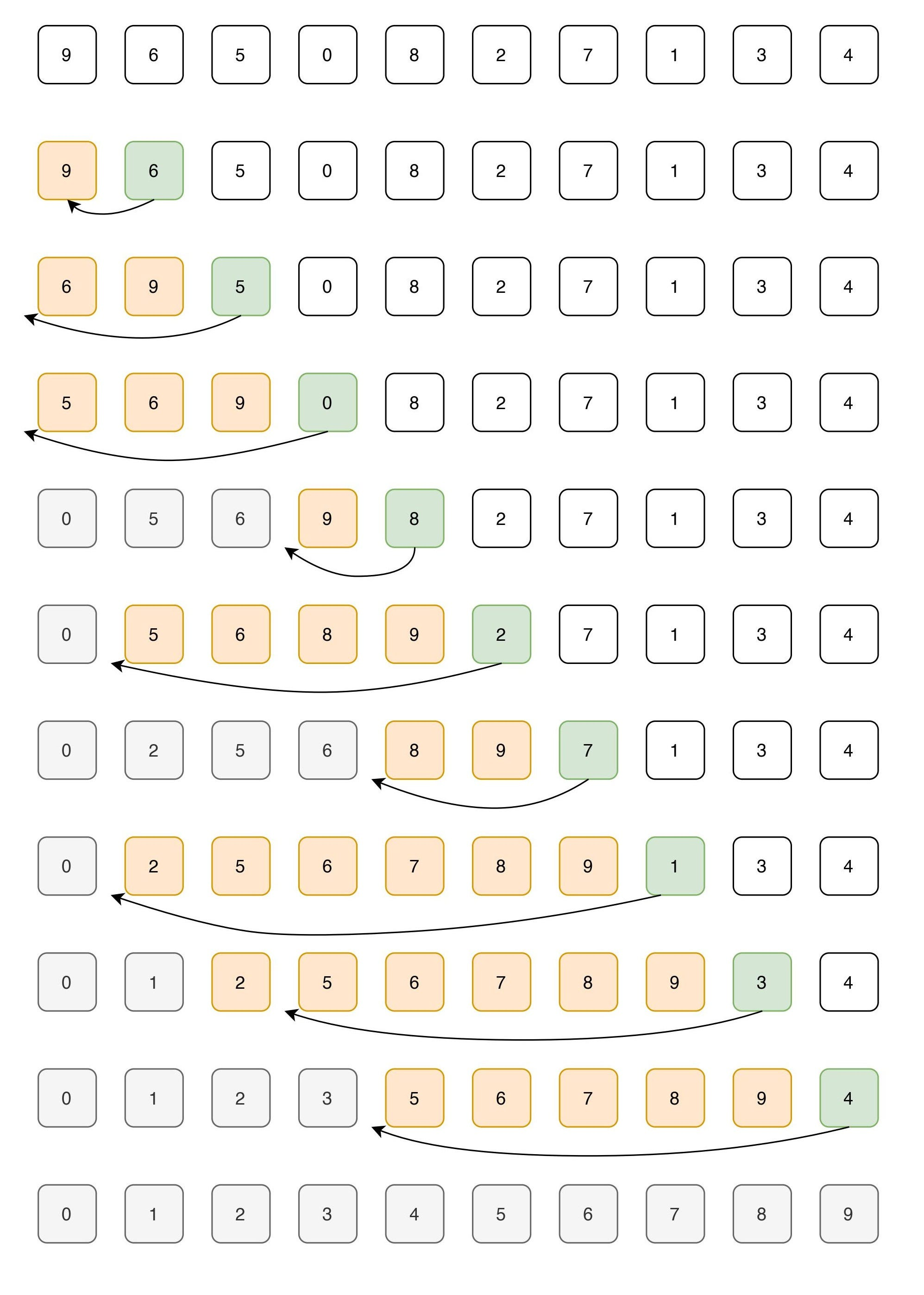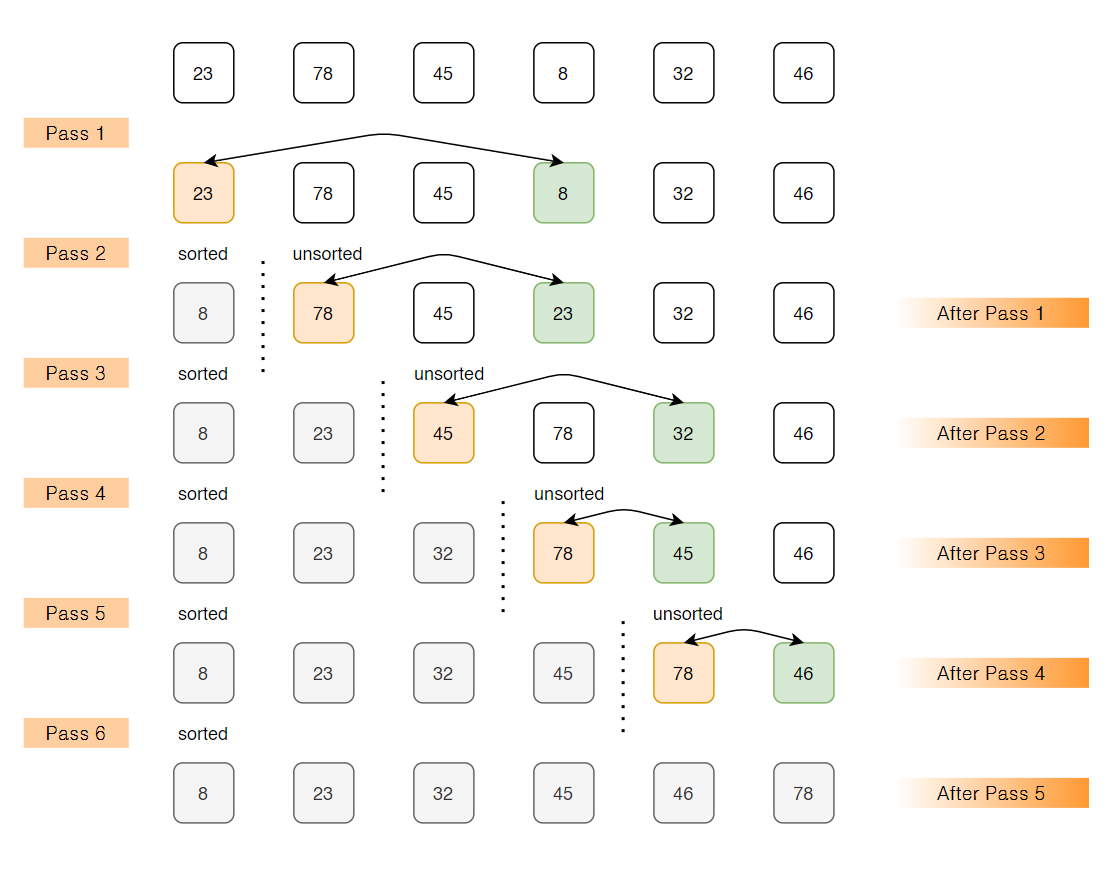# 5 Sorting Algorithms Every Programmer Should Know5 Sorting Algorithms Every Programmer Should Know: Insertion Sort, Selection Sort, Bubble Sort, Merge Sort and Quick Sort. A sorting algorithm is used to rearrange a given array or list of elements as per the comparison operator on the element. Mainly there are five basic algorithms used and you can derive multiple algorithms using these basic algorithms. Each of these algorithms has some pros and cons and can be chosen effectively depending on the size of data to be handled.

Ever wonder how the products in an Amazon or any other e-commerce website get sorted when you apply filters like low-to-high or high-to-low, or alphabetically? Sorting algorithms play a vital role for such websites where you have an enormous amount of products listed and you have to make customer interactions easy.

A sorting algorithm is used to rearrange a given array or list of elements as per the comparison operator on the element. The comparison operator is used to decide the new order of elements in the respective data structure. Mainly there are five basic algorithms used and you can derive multiple algorithms using these basic algorithms. Each of these algorithms has some pros and cons and can be chosen effectively depending on the size of data to be handled.

1. Insertion Sort
2. Selection Sort
3. Bubble Sort
4. Merge Sort
5. Quick Sort

For a complete Data Structures and Algorithm cheatsheet, fork this GitHub repo.

***

## 1. Insertion Sort

Insertion sort is a simple sorting algorithm that works similarly to the way you sort playing cards in your hands. The array is virtually split into a sorted and an unsorted part. Values from the unsorted part are picked and placed at the correct position in the sorted part. Insertion sort is fast and best suitable either when the problem size is small (because it has low overhead) or when the data is nearly sorted (because it is adaptive).

``````Example:
elements: 9, 6, 5, 0, 8, 2, 7, 1, 3, 4
i       : 0, 1, 2, 3, 4, 5, 6, 7, 8, 9
Let us loop for i = 1 (second element of the array) to 9 (last element of the array)
i=1\. Since 6 is smaller than 9, move 9 and insert 6 before 9
6, 9, 5, 0, 8, 2, 7, 1, 3, 4
i=2\. Since 5 is smaller than 6 and 9, move 5 before 6 and 9
5, 6, 9, 0, 8, 2, 7, 1, 3, 4
i=3\. Since 0 is smaller than 5,6 and 9, move 0 before 5,6,9
0, 5, 6, 9, 8, 2, 7, 1, 3, 4
i=4\. Since 8 is smaller than 9, move 8 before 9
0, 5, 6, 8, 9, 2, 7, 1, 3, 4
i=5\. Since 2 is smaller than 5,6,8 and 9, move 2 before 5,6,8,9
0, 2, 5, 6, 8, 9, 7, 1, 3, 4
i=6\. 0, 2, 5, 6, 7, 8, 9, 1, 3, 4
i=7\. 0, 1, 2, 5, 6, 7, 8, 9, 3, 4
i=8\. 0, 1, 2, 3, 5, 6, 7, 8, 9, 4
i=9\. 0, 1, 2, 3, 4, 5, 6, 7, 8, 9``````Insertion Sort

``````Algorithm:

Insertion-Sort(A)
{
for j=i to A.length
key = A[i];
// insert A[i] into sorted sequence A[1,2,3,..,i-1]
j= i-1;
while (j>0 and A[j]>key)
A[j+1] = A[j]
j= j-1
A[j+1] = key
}``````
``````// C program for insertion sort
#include <math.h>
#include <stdio.h>

/* Function to sort an array using insertion sort*/
void insertionSort(int arr[], int n)
{
int i, key, j;
for (i = 1; i < n; i++)
{
key = arr[i];
j = i - 1;

/* Move elements of arr[0..i-1], that are greater than key, to one position ahead
of their current position */
while (j >= 0 && arr[j] > key)
{
arr[j + 1] = arr[j];
j = j - 1;
}
arr[j + 1] = key;
}
}

// A utility function to print an array of size n
void printArray(int arr[], int n)
{
int i;
for (i = 0; i < n; i++)
printf("%d ", arr[i]);
printf("\n");
}

/* Driver program to test insertion sort */
int main()
{
int arr[] = {12, 11, 13, 5, 6};
int n = sizeof(arr) / sizeof(arr);

insertionSort(arr, n);
printArray(arr, n);

return 0;
}``````

## 2. Selection Sort

The selection sort algorithm sorts an array by repeatedly finding the minimum element (considering ascending order) from the unsorted part and putting it at the beginning. The algorithm maintains two subarrays in a given array:

• The subarray which is already sorted
• Remaining subarray which is unsorted

In every iteration/pass of selection sort, the minimum element (considering ascending order) from the unsorted subarray is picked and moved to the sorted subarray. The selection sort has the property of minimizing the number of swaps. Therefore, it is the best choice when the cost of swapping is high.

``````Example:
arr[]= 23 78 45 8 32 46

Pass 1
// Find the minimum element in arr[0...5] and place it at beginning
8 78 45 23 32 46
Pass 2
// Find the minimum element in arr[1...5] and place it at beginning of arr[1...5]
8 23 45 78 32 46
Pass 3
// Find the minimum element in arr[2...5] and place it at beginning of arr[2...5]
8 23 32 78 45 46
Pass 4
// Find the minimum element in arr[3...5] and place it at beginning of arr[3...5]
8 23 32 45 78 46
Pass 5
// Find the minimum element in arr[4...5] and place it at beginning of arr[4...5]
8 23 32 45 46 78``````Selection Sort

``````Algorithm:

void SelectionSort (int a[], int n)
{
int i,j, temp, min;
for (i=0; i<n-1; i++)
{
min = i;
for (j=i+1; j<n; j++)
if (a[j] < a[min])
{
min = j;
}
temp = a[i];
a[i] = a[min];
a[min] = temp;
}
}``````
``````// C program for implementation of selection sort
#include <stdio.h>

void swap(int *xp, int *yp)
{
int temp = *xp;
*xp = *yp;
*yp = temp;
}

void selectionSort(int arr[], int n)
{
int i, j, min_idx;

// One by one move boundary of unsorted subarray
for (i = 0; i < n - 1; i++)
{
// Find the minimum element in unsorted array
min_idx = i;
for (j = i + 1; j < n; j++)
if (arr[j] < arr[min_idx])
min_idx = j;

// Swap the found minimum element with the first element
swap(&arr[min_idx], &arr[i]);
}
}

/* Function to print an array */
void printArray(int arr[], int size)
{
int i;
for (i = 0; i < size; i++)
printf("%d ", arr[i]);
printf("\n");
}

// Driver program to test above functions
int main()
{
int arr[] = {64, 25, 12, 22, 11};
int n = sizeof(arr) / sizeof(arr);
selectionSort(arr, n);
printf("Sorted array: \n");
printArray(arr, n);
return 0;
}``````

## Data structures and algorithms for interviews

An interview for a coding/software position is incomplete without a coding interview. Here’s an explanation for common problems asked

## Why Data Scientists Should Learn Algorithms and Data Structures?

Understanding concepts such as algorithmic complexity and proper use of data structures will enable you to write more optimal code. I will list a few tools you will have under your tool-belt after taking a typical algorithms course.

## Python Data Structures: Your Starter Kit to Learning Algorithms

Arrays, Linked Lists, Stacks, Queues and Hash Tables. Working with any kind of algorithm starts with learning a set of data structures associated with it.

## Python Data Structures: Your Starter Kit to Learning Algorithms

This is just meant as a friendly introduction to a topic that every computer science and data science program I know off explores in an entire course or a few.

## Data Structures and Algorithms Journey

This particular concept is identified as one of the most important concepts in software engineering, and that became a primary checkpoint for most of the top-level companies.